# Excel Applications for Accounting Principles

## Quiz 8 :Gross Profit Method GPLooking for Accounting Homework Help?

## Quiz 8 :Gross Profit Method GPPROBLEM DATA On September 30, 2013, the general ledger of Leon's Golf Shop, which uses the calendar year as its accounting period, showed the following year-to-date account balances: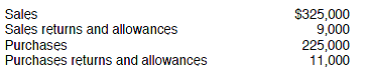The merchandise inventory account had a $48,000 balance on January 1, 2013. The historical gross profit percentage is 40%. REQUIREMENT Leon prepares quarterly financial statements and takes physical inventory once a year-at the end of the accounting period. In order to prepare the financial statements for the third quarter, the store needs to have an estimate of ending inventory. You have been asked to use the gross profit method to estimate the ending inventory. Review the worksheet called GP. Study it carefully because it may have a solution format somewhat different from the one shown in your textbook. Free Essay Answer: Answer: The worksheet to estimate ending inventory using the gross profit method is shown below: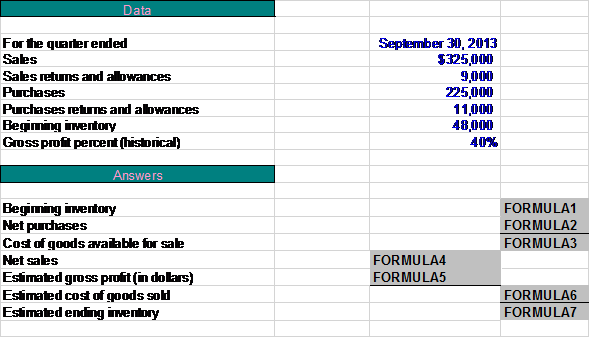PROBLEM DATA On September 30, 2013, the general ledger of Leon's Golf Shop, which uses the calendar year as its accounting period, showed the following year-to-date account balances:The merchandise inventory account had a$48,000 balance on January 1, 2013. The historical gross profit percentage is 40%. REQUIREMENT Open the file GP from the website for this book at cengagebrain.com. Enter all the formulas where indicated on the worksheet. Enter your name in cell A1. Save your completed worksheet as GP2. Print the worksheet. Also print your formulas. Check figure: Estimated ending inventory (cell D23), $72,400. Free Essay Answer: Answer: The formulas in the worksheet will be entered using cell references when appropriate. Net purchases are calculated by deducting purchases returns and allowances from purchases. Cost of goods available for sale is the total of beginning inventory and net purchases. Net sales are calculated by deducting sales returns and allowances from sales. Gross profit is determined by the historical gross profit percentage of net sales. If gross profit percentage is 40% then we can assume that cost of goods sold is 60% of net sales. Estimated ending inventory is cost of goods available for sales less estimated cost of goods sold. The required worksheet is shown below: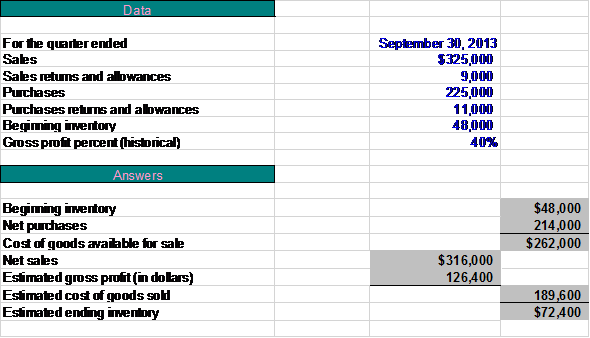WHAT-IF ANALYSIS On December 31, 2013, the year-to-date account balances of selected accounts were as follows: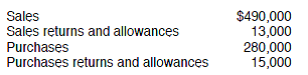Estimated ending merchandise inventory at December 31, 2013, is$ __________.
Free
Essay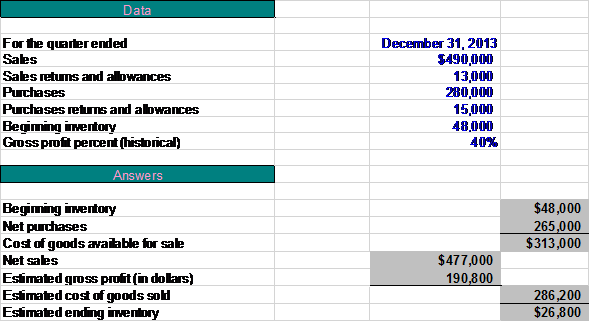The estimated ending inventory on December 21, 2013 is $26,800.WHAT-IF ANALYSIS A physical count of merchandise inventory on December 31, 2013, revealed inventory costing$22,000. In the space below, list at least two possible reasons for this balance to be different from the estimate computed in requirement 3.